# BCD to Seven Segment Display Decoder Theory

The Seven segment display is most frequently used the digital display in calculators, digital counters, digital clocks, measuring instruments, etc. Usually, the displays like LED’s as well as LCD’s are used to display the characters as well as numerical numbers. But, a seven segment display is used to display both the numbers and characters. These displays are frequently driven by the output phases of digital integrated circuits like decade counters as well as latches. However the outputs of these are in the type of 4-bit BCD (Binary Coded Decimal), so not appropriate for directly operating the seven segment display. For that, a display decoder can be employed for converting BCD code to seven segment code. Generally, it has four input lines as well as seven output lines. This article discusses how to design a BCD to seven segment display decoder circuit using logic gates.

## BCD to Seven Segment Display Decoder Theory

The decoder is an essential component in BCD to seven segment decoder. A decoder is nothing but a combinational logic circuit mainly used for converting a BCD to an equivalent decimal number. It can be a BCD to seven segment decoder. A combinational logic circuit can be built with logic gates which include inputs as well as outputs. The output of this circuit mainly lies in the current condition of the inputs. The best examples of this circuit are multiplexers, demultiplexers, adders, subtractors, encoders, decoders, etc.

The circuit design, as well as operation, mainly depends on the concepts of Boolean algebra as well as logic gates. A seven segment LED display circuit can be built with eight LEDs. The common terminals are either anode otherwise cathode. A general cathode seven segment display includes 8 pins where 7-pins are input pins that are marked with from a to g & 8th-pin is a ground pin.

## Design of BCD to 7 Segment Display Decoder Circuit

The designing of BCD to seven segment display decoder circuit mainly involves four steps namely analysis, truth table design, K-map and designing a combinational logic circuit using logic gates.

The first step of this circuit design is an analysis of the common cathode seven segment display. This display can be constructed with seven LEDs in the form of H. A truth table of this circuit can be designed by the inputs combinations for every decimal digit. For instance, decimal number ‘1’ would control a blend of b & c.

The second step is the truth table design by listing the display input signals-7, equivalent four-digit binary numbers as well as decimal number.

The designing of the truth table for the decoder mainly depends on the kind of display. Already we have discussed above that is, for a common cathode display, the decoder output must be high in order to blink the segment.

The tabular form of a BCD to 7-segment decoder with a common cathode display is shown below. The truth table consists of seven o/p columns equivalent to each of the seven segments. For example, the column for a-segment illustrates the various arrangements for which it is to be light up. Thus ‘a’- segment is energetic for the digits like 0, 2, 3, 5, 6, 7, 8 & 9.

 Digit X Y Z W a b c d e f g 0 0 0 0 0 0 0 0 0 0 0 1 1 0 0 0 1 1 0 0 1 1 1 1 2 0 0 1 0 0 0 1 0 0 1 0 3 0 0 1 1 0 0 0 0 1 1 0 4 0 1 0 0 1 0 0 1 1 0 0 5 0 1 0 1 0 1 0 0 1 0 0 6 0 1 1 0 0 1 0 0 0 0 0 7 0 1 1 1 0 0 0 1 1 1 1 8 1 0 0 0 0 0 0 0 0 0 0 9 1 0 0 1 0 0 0 0 1 0 0

By using the above truth table, for every output function, the Boolean expression can be written.

a = F1 (X, Y, Z, W) = ∑m (0, 2, 3, 5, 7, 8, 9)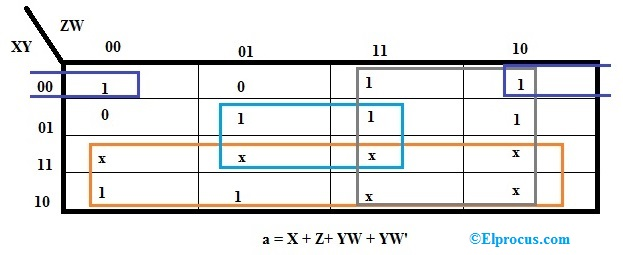b = F2 (X, Y, Z, W) = ∑m (0, 1, 2, 3, 4, 7, 8, 9)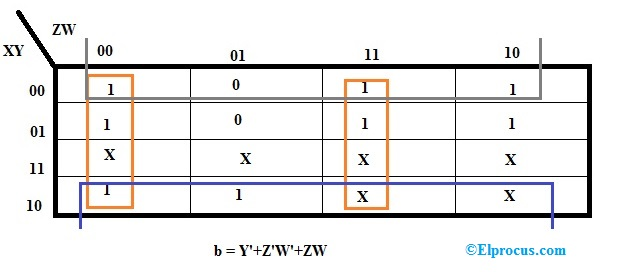c = F3 (X, Y, Z, W) = ∑m (0, 1, 3, 4, 5, 6, 7, 8, 9)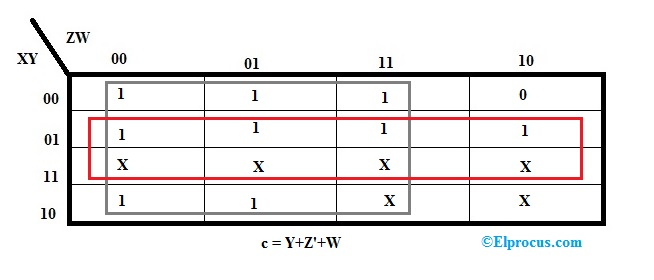d = F4 (X, Y, Z, W) = ∑m (0, 2, 3, 5, 6, 8)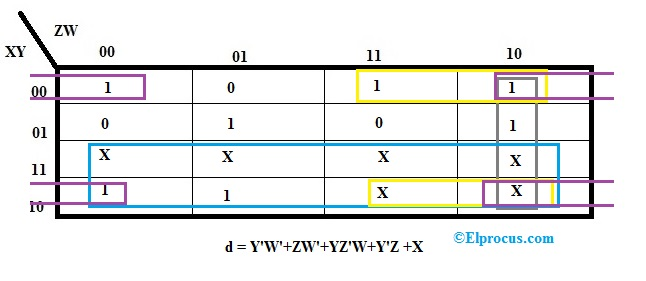e = F5 (X, Y, Z, W) = ∑m (0, 2, 6, 8)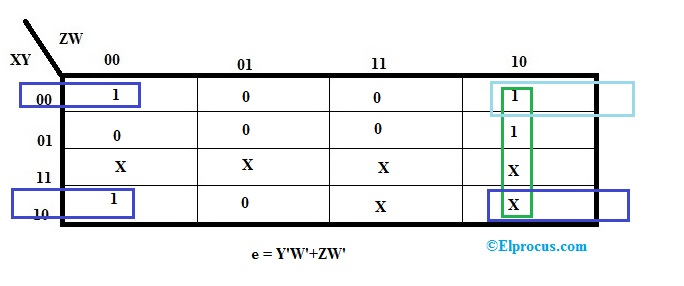f = F6 (X, Y, Z, W) = ∑m (0, 4, 5, 6, 8, 9)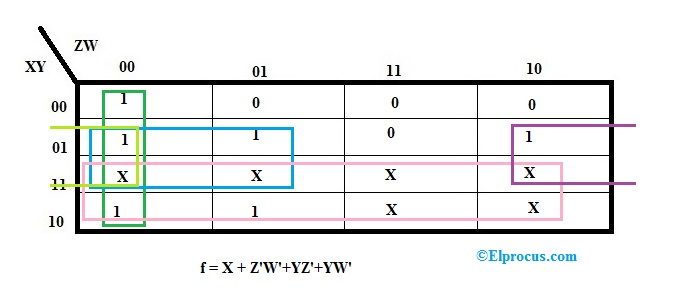g = F7 (X, Y, Z, W) = ∑m (2, 3, 4, 5, 6, 8, 9)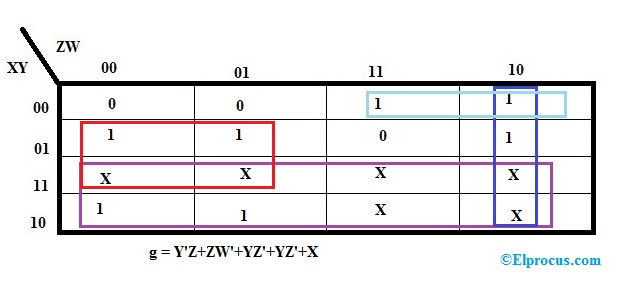The third step in this design mainly involves designing the K-map (Karnaugh’s map) for every output expression as well as then shortening them to get inputs logic combination for every output.

### Simplification of Karnaugh -Map

The simplification of k-map of the common cathode 7 segment decoder can be done in order to plan the combinational circuit. From the above K-map simplification, we can get the output equations like these

a = X+Z+YW+Y’W’

b = Y’+Z’W’+ZW

c= Y+Z’+W

d = Y’W’+ZW’+YZ’W+Y’Z+X

e= Y’W’+ZW’

f= X + Z’W’+YZ’+YW’

g = X+YZ’+Y’Z+ZW’

The final step of this is a designing of a logic circuit using the above k-map equations. A combinational circuit can be built by using 4-inputs namely A, B, C, D and outputs on display like a, b, c, d, e, f, g. The operation of the above logic circuit can be understood with the help of truth table only. Once all the i/ps are connected to small logic.